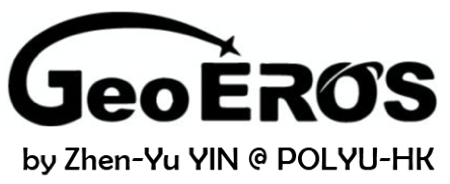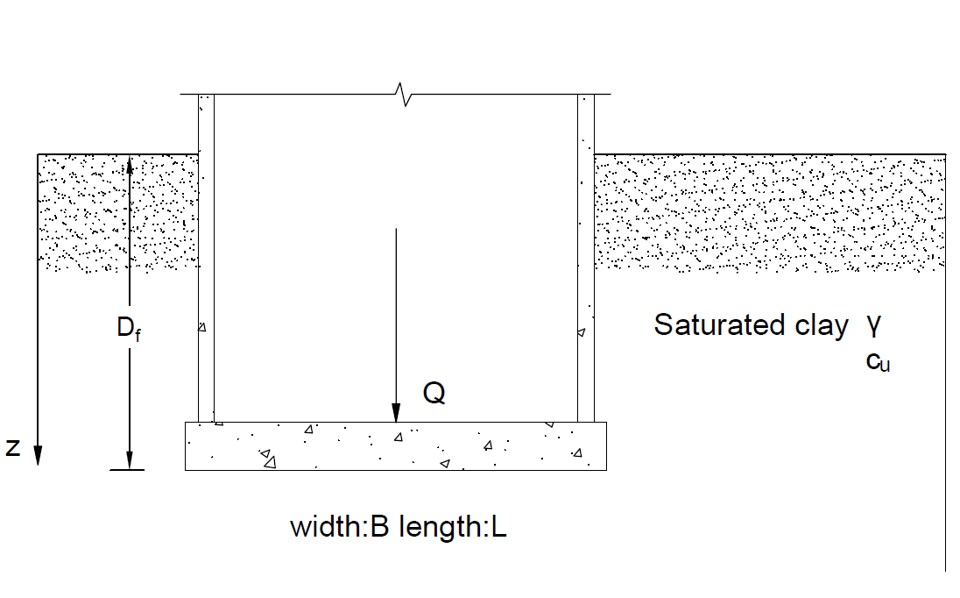Mat Foundation Tool in Clay (qu)Geometry of Mat Foundation in Clay (bearing capacity)Tips

• This tool is for evaluating bearing capacity of mat foundation in clay, as shown in the figure above.
• All the input parameters should be positive.
• The unit weight of water is 9.8 kN/m3.
• For "nan", "0" or "inf" displayed in Results, please check your input parameters.
• If Q is given to estimate Fs, users can choose "Input Q to find Fs". If Fs is given to estimate Q, users can choose "Input Fs to find Q".
• Trial-error process can be used to estimate the dimension of the foundation (width,length and depth) for a given Q and a required Fs.

B (m)
L (m)
Df (m)
γ (kN/m3)
cu (kN/m2)